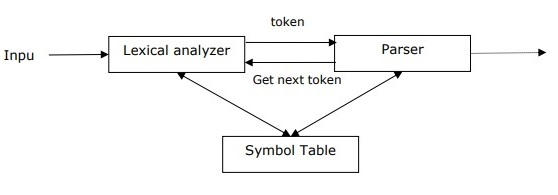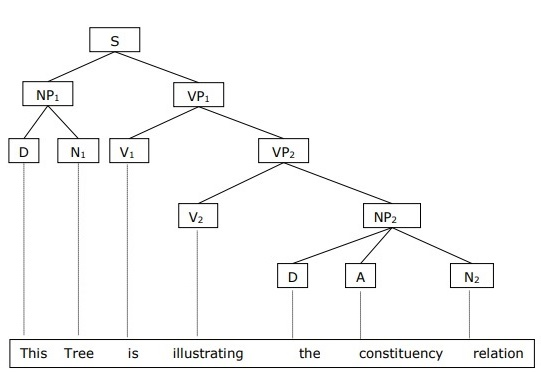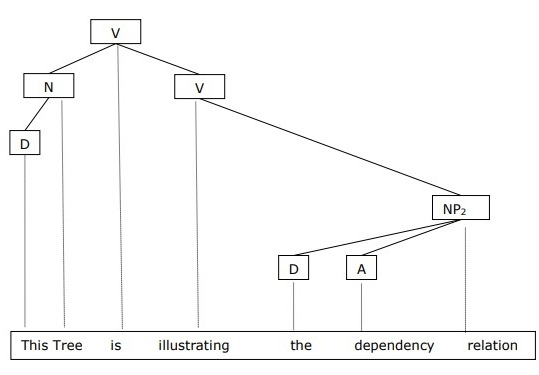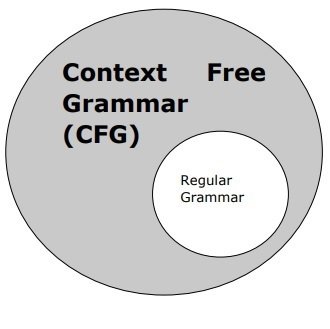# Natural Language Processing - Syntactic Analysis

Syntactic analysis or parsing or syntax analysis is the third phase of NLP. The purpose of this phase is to draw exact meaning, or you can say dictionary meaning from the text. Syntax analysis checks the text for meaningfulness comparing to the rules of formal grammar. For example, the sentence like “hot ice-cream” would be rejected by semantic analyzer.

In this sense, syntactic analysis or parsing may be defined as the process of analyzing the strings of symbols in natural language conforming to the rules of formal grammar. The origin of the word ‘parsing’ is from Latin word ‘pars’ which means ‘part’.

## Concept of Parser

It is used to implement the task of parsing. It may be defined as the software component designed for taking input data (text) and giving structural representation of the input after checking for correct syntax as per formal grammar. It also builds a data structure generally in the form of parse tree or abstract syntax tree or other hierarchical structure.The main roles of the parse include −

• To report any syntax error.

• To recover from commonly occurring error so that the processing of the remainder of program can be continued.

• To create parse tree.

• To create symbol table.

• To produce intermediate representations (IR).

## Types of Parsing

Derivation divides parsing into the followings two types −

• Top-down Parsing

• Bottom-up Parsing

### Top-down Parsing

In this kind of parsing, the parser starts constructing the parse tree from the start symbol and then tries to transform the start symbol to the input. The most common form of topdown parsing uses recursive procedure to process the input. The main disadvantage of recursive descent parsing is backtracking.

### Bottom-up Parsing

In this kind of parsing, the parser starts with the input symbol and tries to construct the parser tree up to the start symbol.

## Concept of Derivation

In order to get the input string, we need a sequence of production rules. Derivation is a set of production rules. During parsing, we need to decide the non-terminal, which is to be replaced along with deciding the production rule with the help of which the non-terminal will be replaced.

## Types of Derivation

In this section, we will learn about the two types of derivations, which can be used to decide which non-terminal to be replaced with production rule −

### Left-most Derivation

In the left-most derivation, the sentential form of an input is scanned and replaced from the left to the right. The sentential form in this case is called the left-sentential form.

### Right-most Derivation

In the left-most derivation, the sentential form of an input is scanned and replaced from right to left. The sentential form in this case is called the right-sentential form.

## Concept of Parse Tree

It may be defined as the graphical depiction of a derivation. The start symbol of derivation serves as the root of the parse tree. In every parse tree, the leaf nodes are terminals and interior nodes are non-terminals. A property of parse tree is that in-order traversal will produce the original input string.

## Concept of Grammar

Grammar is very essential and important to describe the syntactic structure of well-formed programs. In the literary sense, they denote syntactical rules for conversation in natural languages. Linguistics have attempted to define grammars since the inception of natural languages like English, Hindi, etc.

The theory of formal languages is also applicable in the fields of Computer Science mainly in programming languages and data structure. For example, in ‘C’ language, the precise grammar rules state how functions are made from lists and statements.

A mathematical model of grammar was given by Noam Chomsky in 1956, which is effective for writing computer languages.

Mathematically, a grammar G can be formally written as a 4-tuple (N, T, S, P) where −

• N or VN = set of non-terminal symbols, i.e., variables.

• T or = set of terminal symbols.

• S = Start symbol where S ∈ N

• P denotes the Production rules for Terminals as well as Non-terminals. It has the form α → β, where α and β are strings on VN ∪ ∑ and least one symbol of α belongs to VN

## Phrase Structure or Constituency Grammar

Phrase structure grammar, introduced by Noam Chomsky, is based on the constituency relation. That is why it is also called constituency grammar. It is opposite to dependency grammar.

### Example

Before giving an example of constituency grammar, we need to know the fundamental points about constituency grammar and constituency relation.

• All the related frameworks view the sentence structure in terms of constituency relation.

• The constituency relation is derived from the subject-predicate division of Latin as well as Greek grammar.

• The basic clause structure is understood in terms of noun phrase NP and verb phrase VP.

We can write the sentence “This tree is illustrating the constituency relation” as follows −## Dependency Grammar

It is opposite to the constituency grammar and based on dependency relation. It was introduced by Lucien Tesniere. Dependency grammar (DG) is opposite to the constituency grammar because it lacks phrasal nodes.

### Example

Before giving an example of Dependency grammar, we need to know the fundamental points about Dependency grammar and Dependency relation.

• In DG, the linguistic units, i.e., words are connected to each other by directed links.

• The verb becomes the center of the clause structure.

• Every other syntactic units are connected to the verb in terms of directed link. These syntactic units are called dependencies.

We can write the sentence “This tree is illustrating the dependency relation” as follows;Parse tree that uses Constituency grammar is called constituency-based parse tree; and the parse trees that uses dependency grammar is called dependency-based parse tree.

## Context Free Grammar

Context free grammar, also called CFG, is a notation for describing languages and a superset of Regular grammar. It can be seen in the following diagram −## Definition of CFG

CFG consists of finite set of grammar rules with the following four components −

### Set of Non-terminals

It is denoted by V. The non-terminals are syntactic variables that denote the sets of strings, which further help defining the language, generated by the grammar.

### Set of Terminals

It is also called tokens and defined by Σ. Strings are formed with the basic symbols of terminals.

### Set of Productions

It is denoted by P. The set defines how the terminals and non-terminals can be combined. Every production(P) consists of non-terminals, an arrow, and terminals (the sequence of terminals). Non-terminals are called the left side of the production and terminals are called the right side of the production.

### Start Symbol

The production begins from the start symbol. It is denoted by symbol S. Non-terminal symbol is always designated as start symbol.